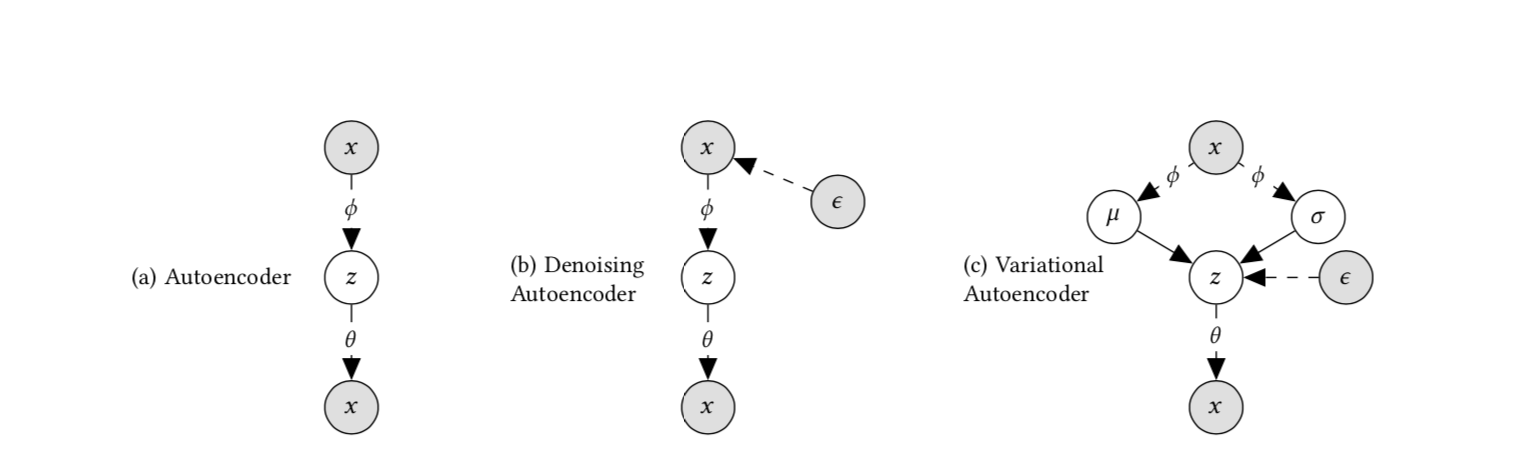# MultiVAE¶

## Introduction¶

[paper]

Title: Variational Autoencoders for Collaborative Filtering

Authors: Dawen Liang, Rahul G, Matthew D Hoffman, Tony Jebara

Abstract: We extend variational autoencoders (VAEs) to collaborative filtering for implicit feedback. This non-linear probabilistic model enables us to go beyond the limited modeling capacity of linear factor models which still largely dominate collaborative filtering research.We introduce a generative model with multinomial likelihood and use Bayesian inference for parameter estimation. Despite widespread use in language modeling and economics, the multinomial likelihood receives less attention in the recommender systems literature. We introduce a different regularization parameter for the learning objective, which proves to be crucial for achieving competitive performance. Remarkably, there is an efficient way to tune the parameter using annealing. The resulting model and learning algorithm has information-theoretic connections to maximum entropy discrimination and the information bottleneck principle. Empirically, we show that the proposed approach significantly outperforms several state-of-the-art baselines, including two recently-proposed neural network approaches, on several real-world datasets. We also provide extended experiments comparing the multinomial likelihood with other commonly used likelihood functions in the latent factor collaborative filtering literature and show favorable results. Finally, we identify the pros and cons of employing a principled Bayesian inference approach and characterize settings where it provides the most significant improvements.## Running with RecBole¶

Model Hyper-Parameters:

• `latent_dimendion (int)` : The latent dimension of auto-encoder. Defaults to `128`.

• `mlp_hidden_size (list)` : The MLP hidden layer. Defaults to ``.

• `dropout_prob (float)` : The drop out probability of input. Defaults to `0.5`.

• `anneal_cap (float)` : The hyper parameter of the weight of KL loss. Defaults to `0.2`.

• `total_anneal_steps (int)` : The maximum steps of anneal update. Defaults to `200000`.

A Running Example:

Write the following code to a python file, such as run.py

```from recbole.quick_start import run_recbole

parameter_dict = {
'train_neg_sample_args': None,
}
run_recbole(model='MultiVAE', dataset='ml-100k', config_dict=parameter_dict)
```

And then:

```python run.py
```

Note: Because this model is a non-sampling model, so you must set `train_neg_sample_args=None` when you run this model.

## Tuning Hyper Parameters¶

If you want to use `HyperTuning` to tune hyper parameters of this model, you can copy the following settings and name it as `hyper.test`.

```learning_rate choice [0.01,0.005,0.001,0.0005,0.0001]
```

Note that we just provide these hyper parameter ranges for reference only, and we can not guarantee that they are the optimal range of this model.

Then, with the source code of RecBole (you can download it from GitHub), you can run the `run_hyper.py` to tuning:

```python run_hyper.py --model=[model_name] --dataset=[dataset_name] --config_files=[config_files_path] --params_file=hyper.test
```

For more details about Parameter Tuning, refer to Parameter Tuning.

If you want to change parameters, dataset or evaluation settings, take a look at